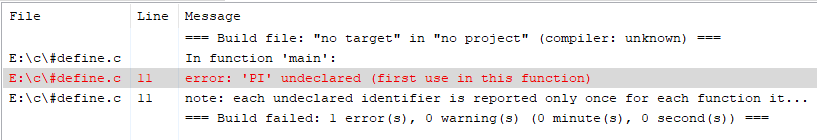# #define vs #undef in C language

• Difficulty Level : Basic
• Last Updated : 04 May, 2021

In this article, we will discuss the difference between #define and #undef pre-processor in C language.

Pre-Processor:

• Pre-processor is a program that performs before the compilation.
• It only notices the # started statement.
• # is called preprocessor directive.
• Each preprocessing directive must be on its own line.
• The word after # is called the preprocessor command.

#define:

The #define directive defines an identifier and a character sequence (a set of characters) that will be substituted for the identifier each time it is encountered in the source file.

Syntax:

#define macro-name char-sequence.
The identifier is referred to as a macro name and replacement process as a macro replacement.

Example:

#define PI 3.14
Here. PI is the macro-name and 3.14 is the char-sequence.

Program 1:

Below is the C program illustrating the use of #define:

## C

 `// C program illustrating the use of ` `// #define ` `#include ` `#define PI 3.14 ` ` `  `// Driver Code ` `int` `main() ` `{ ` `    ``int` `r = 4; ` `    ``float` `a; ` ` `  `    ``a = PI * r * r; ` `    ``printf``(``"area of circle is %f"``, a); ` ` `  `    ``return` `0; ` `} `

Output:

```area of circle is 50.240002
```

Explanation:

• In this example, PI is the macro-name and the char-sequence is 3.14.
• When the program runs the compiler will check the #define command first and assign the PI as 3.14.
• Now in the entire program wherever the compiler sees the PI word it will replace it with 3.14.

Program 2:

Below is the C program printing product of two numbers using #define:

## C

 `// C program to find the product of ` `// two numbers using #define ` ` `  `#include ` `#define PRODUCT(a, b) a* b ` ` `  `// Driver Code ` `int` `main() ` `{ ` `    ``printf``(``"product of a and b is "` `           ``"%d"``, ` `           ``PRODUCT(3, 4)); ` ` `  `    ``return` `0; ` `} `

Output:

```product of a and b is 12
```

Explanation:

• In this example, a macro-name as the product is defined and passes two arguments as a and b and gives the char-sequence as the product of these two arguments.
• When the compiler sees the macro-name in the print statement, it replaces the macro-name with the product of a and b and gives the answer as their product.

#undef:

The #undef preprocessor directive is used to undefined macros.

Syntax:

#undef macro-name

Program 3:

Below is the C program to illustrate the use of #undef in a program:

## C

 `// C program to illustrate the use ` `// of #undef in a program ` `#include ` `#define PI 3.14 ` `#undef PI ` ` `  `// Driver Code ` `int` `main() ` `{ ` `    ``int` `r = 6; ` `    ``float` `a; ` `    ``a = PI * r * r; ` ` `  `    ``printf``(``"area of circle is %f"``, a); ` ` `  `    ``return` `0; ` `}`

Output:Explanation: In this example, when #undef is used, then it will delete the #define command and the macro will get undefined and the compiler will show the error.

My Personal Notes arrow_drop_up
Recommended Articles
Page :